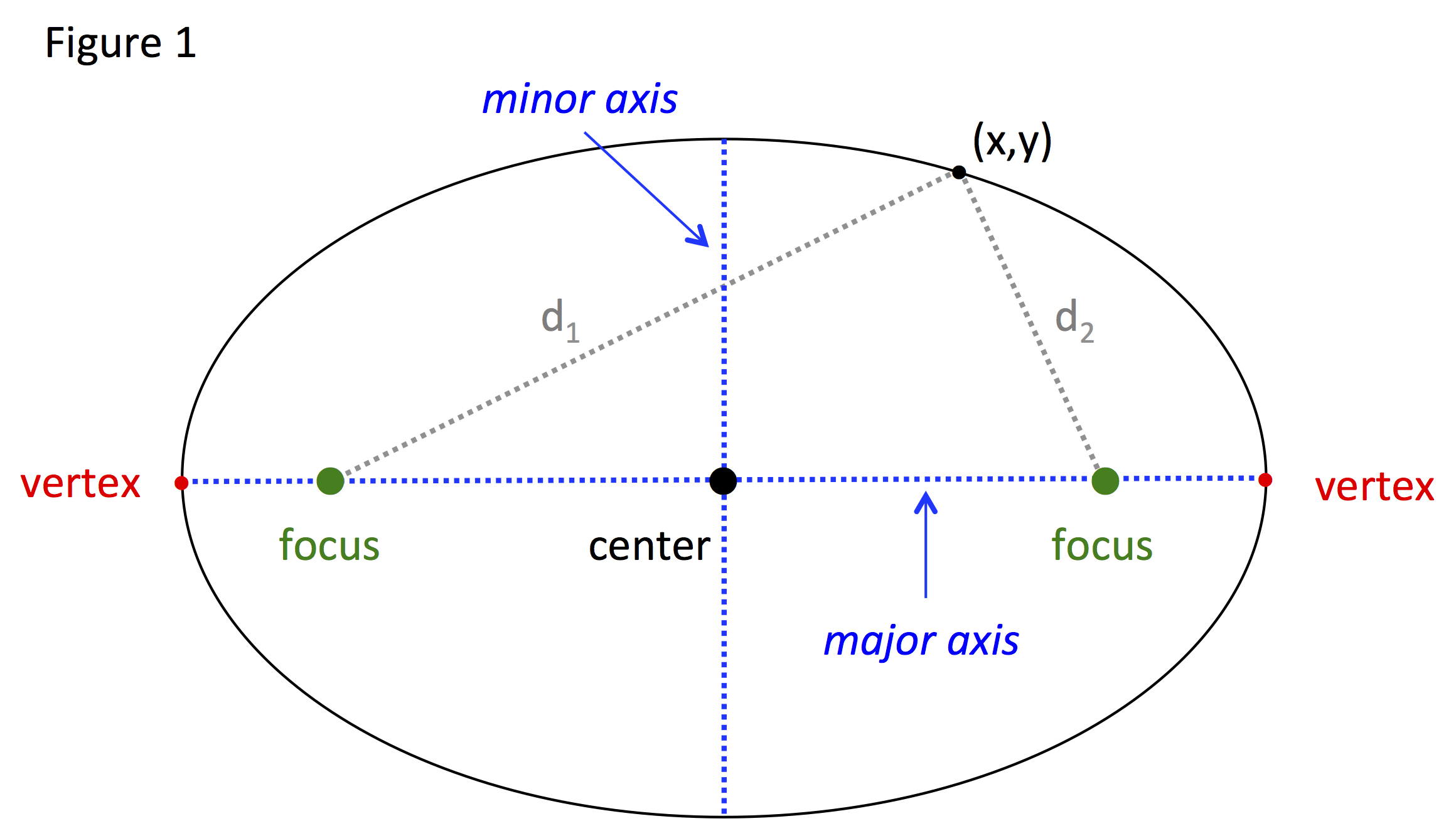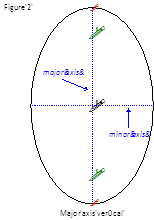# Ellipse: Standard Equation

An ellipse is a conic section that is described as:

DEFINITION OF AN ELLIPSE:

The set of all points (x, y) in a plane the sum of whose distances from two fixed points, called foci, is constant.

Figure 1 shows a picture of an ellipse:Thus for all (x, y), d1 + d2 = constant. When talking about an ellipse, the following terms are used:

• The foci are two fixed points equidistant from the center of the ellipse.

• The vertices are the points on the ellipse that fall on the line containing the foci.

• The line segment or chord joining the vertices is the major axis.

• The midpoint of the major axis is the center.

• The axis perpendicular to the major axis is the minor axis.

Figure 1 shows an ellipse with a horizontal major axis. However an ellipse could also have a major axis that is vertical as shown in Figure 2.The standard equation of an ellipse is:

STANDARD EQUATION OF AN ELLIPSE:

➢ Center coordinates (h, k)

➢ Major axis 2a

➢ Major axis 2b

➢ 0 < b < a

$\frac{{\left(x-h\right)}^{2}}{{a}^{2}}+\frac{{\left(y-k\right)}^{2}}{{b}^{2}}=1$ major axis is horizontal

$\frac{{\left(x-h\right)}^{2}}{{b}^{2}}+\frac{{\left(y-k\right)}^{2}}{{a}^{2}}=1$ major axis is vertical

The foci lie on the major axis, c units from the center, with c2 = a2 - b2.

Let's use these equations in some examples:

Example 1: Find the standard equation of the ellipse having foci at (2, 1) and (-4, 1) and a minor axis of 10.
 Step 1: Determine the following: ➢ The coordinates of the center (h, k). ➢ The distance of half the minor axis (b). ➢ The length of half the major axis (a). ➢ The orientation of the major axis. Center: Since the foci are equidistant from the center of the ellipse the center can be determine by finding the midpoint of the foci. Length of b: The minor axis is given as 10, which is equal to 2b. $2b=10\to {b}={5}$ Length of a: To find a the equation c2 = a2 + b2 can be used but the value of c must be determined. Since c is the distance from the foci to the center, take either foci and determine the distance to the center. Then solve for a. Foci (2, 1): $c=|2-\left(-1\right)|=|3|=3$ Foci (-4, 1): c = 3 ${c}^{2}={a}^{2}-{b}^{2}\to a=\sqrt{{c}^{2}+{b}^{2}}$ $a=\sqrt{{{3}}^{2}+{{5}}^{2}}\to {a}{=}\sqrt{34}$ Orientation of major axis: Since the two foci fall on the horizontal line y = 1, the major axis is horizontal. Step 2: Substitute the values for h, k, a and b into the equation for an ellipse with a horizontal major axis. Horizontal major axis equation: $\frac{{\left(x-h\right)}^{2}}{{a}^{2}}+\frac{{\left(y-k\right)}^{2}}{{b}^{2}}=1$ Substitute values: $\frac{{\left[x-\left(-1\right)\right]}^{2}}{{\sqrt{34}}^{2}}+\frac{{\left(y-1\right)}^{2}}{{{5}}^{2}}=1$ Simplify: $\frac{{\left(x+1\right)}^{2}}{{\sqrt{34}}^{2}}+\frac{{\left(y-1\right)}^{2}}{{5}^{2}}=1$
Example 2: Find the standard equation of an ellipse represented by x2 + 3y2 - 4x - 18y + 4 = 0.
 Step 1: Group the x- and y-terms on the left-hand side of the equation. x2 + 3y2 - 4x - 18y + 4 = 0 $\left({x}^{2}-4x\right)+\left(3{y}^{2}-18y\right)+4=0$ Step 2: Move the constant term to the right-hand side. $\left({x}^{2}-4x\right)+\left(3{y}^{2}-18y\right)=-4$ Step 3: Complete the square for the x- and y-groups. $\left({x}^{2}-4x\right)+\left(3{y}^{2}-18y\right)=-4$ Complete the square for the x-group (x2 - 4x) Take the coefficient of the x-term, divide by 2 and square the result. $\frac{-4}{2}=-2;{\left(-2\right)}^{2}=4$ Add the result to the x-group. (x2 - 4x + 4) Complete the square for the y-group (3y2 - 18y) Factor out a 3 so they y2-coefficient is 1 3(y2 - 6y) Take the coefficient of the y-term, divide by 2 and square the result. $\frac{-6}{2}=-3;{\left(-3\right)}^{2}=9$ Add the result to the y-group. 3(y2 - 6y + 9) Final result. $\left({x}^{2}-4x+4\right)+3\left({y}^{2}-6y+9\right)=-4+?$ Step 4: Add whatever was added to the left-hand side to the right-hand side. $\left({x}^{2}-4x+4\right)+3\left({y}^{2}-6y+9\right)=-4+4+3\left(9\right)$ $\left({x}^{2}-4x+4\right)+3\left({y}^{2}-6y+9\right)=27$ Step 5: Write the x-group and y-group as perfect squares. ${\left(x-2\right)}^{2}+3{\left(y-3\right)}^{2}=27$ Step 6: Divide both sides by the value on the right-hand side. $\frac{{\left(x-2\right)}^{2}}{27}+\frac{3{\left(y-3\right)}^{2}}{27}=1$ $\frac{{\left(x-2\right)}^{2}}{27}+\frac{{\left(y-3\right)}^{2}}{9}=1$

 Related Links: Math algebra Ellipse: Eccentricity Hyperbola: Standard Equation Pre Calculus

To link to this Ellipse: Standard Equation page, copy the following code to your site: